International Tables for Crystallography (2006). Vol. D, ch. 3.4, pp. 449-505https://doi.org/10.1107/97809553602060000645

## Contents

• 3.4. Domain structures  (pp. 449-505)
• 3.4.1. Introduction  (pp. 449-451) | html | pdf |
• 3.4.1.1. Basic concepts  (pp. 449-450) | html | pdf |
• 3.4.1.2. Scope of this chapter  (pp. 450-451) | html | pdf |
• 3.4.2. Domain states  (pp. 451-470) | html | pdf |
• 3.4.2.1. Principal and basic domain states  (pp. 451-455) | html | pdf |
• 3.4.2.2. Secondary domain states, partition of domain states  (pp. 455-457) | html | pdf |
• 3.4.2.2.1. Ferroelastic domain state  (pp. 455-457) | html | pdf |
• 3.4.2.2.2. Ferroelectric domain states  (p. 457) | html | pdf |
• 3.4.2.2.3. Domain states with the same stabilizer  (p. 457) | html | pdf |
• 3.4.2.3. Property tensors associated with ferroic domain states  (pp. 458-460) | html | pdf |
• 3.4.2.4. Synoptic table of ferroic transitions and domain states  (pp. 460-462) | html | pdf |
• 3.4.2.4.1. Explanation of Table 3.4.2.7  (pp. 461-462) | html | pdf |
• 3.4.2.5. Basic (microscopic) domain states and their partition into translation subsets  (pp. 462-470) | html | pdf |
• 3.4.3. Domain pairs: domain twin laws, distinction of domain states and switching  (pp. 470-491) | html | pdf |
• 3.4.3.1. Domain pairs and their symmetry, twin law  (pp. 470-473) | html | pdf |
• 3.4.3.2. Twinning group, distinction of two domain states  (pp. 473-475) | html | pdf |
• 3.4.3.3. Switching of ferroic domain states  (pp. 475-476) | html | pdf |
• 3.4.3.4. Classes of equivalent domain pairs and their classifications  (pp. 476-477) | html | pdf |
• 3.4.3.5. Non-ferroelastic domain pairs: twin laws, domain distinction and switching fields, synoptic table  (pp. 477-480) | html | pdf |
• 3.4.3.5.1. Explanation of Table 3.4.3.4  (pp. 477-480) | html | pdf |
• 3.4.3.6. Ferroelastic domain pairs  (pp. 480-491) | html | pdf |
• 3.4.3.6.1. Spontaneous strain  (pp. 480-481) | html | pdf |
• 3.4.3.6.2. Equally deformed planes of a ferroelastic domain pair  (pp. 481-483) | html | pdf |
• 3.4.3.6.3. Disoriented domain states, ferroelastic domain twins and their twin laws  (pp. 483-486) | html | pdf |
• 3.4.3.6.4. Ferroelastic domain pairs with compatible domain walls, synoptic table  (pp. 486-490) | html | pdf |
• 3.4.3.6.4.1. Explanation of Table 3.4.3.6  (pp. 486-490) | html | pdf |
• 3.4.3.6.5. Ferroelastic domain pairs with no compatible domain walls, synoptic table  (pp. 490-491) | html | pdf |
• 3.4.3.7. Domain pairs in the microscopic description  (p. 491) | html | pdf |
• 3.4.4. Domain twins and domain walls  (pp. 491-502) | html | pdf |
• 3.4.4.1. Formal description of simple domain twins and planar domain walls of zero thickness  (pp. 491-492) | html | pdf |
• 3.4.4.2. Layer groups  (pp. 492-493) | html | pdf |
• 3.4.4.3. Symmetry of simple twins and planar domain walls of zero thickness  (pp. 493-496) | html | pdf |
• 3.4.4.4. Non-ferroelastic domain twins and domain walls  (pp. 496-498) | html | pdf |
• 3.4.4.5. Ferroelastic domain twins and walls. Ferroelastic twin laws  (pp. 498-499) | html | pdf |
• 3.4.4.6. Domain walls of finite thickness – continuous description  (pp. 499-501) | html | pdf |
• 3.4.4.7. Microscopic structure and symmetry of domain walls  (pp. 501-502) | html | pdf |
• 3.4.5. Glossary  (pp. 502-503) | html | pdf |
• References | html | pdf |
• Figures
• Fig. 3.4.1.1. Domain structure of tetragonal barium titanate (BaTiO 3 )  (p. 449) | html | pdf |
• Fig. 3.4.1.2. Domain structure of a BaGa 2 O 4 crystal seen by high-resolution transmission electron microscopy  (p. 449) | html | pdf |
• Fig. 3.4.1.3. Elastic hysteresis of ferroelastic lead phosphate Pb 3 (PO 4 ) 2 (Salje, 1990)  (p. 450) | html | pdf |
• Fig. 3.4.1.4. Transmission electron microscopy image of the ferroelastic domain structure in a YBa 2 Cu 3 O 7− y crystal  (p. 450) | html | pdf |
• Fig. 3.4.1.5. Non-ferroelastic ferroelectric domains in triglycine sulfate (TGS) revealed by a liquid-crystal method  (p. 450) | html | pdf |
• Fig. 3.4.2.1. Hierarchy in domain-structure analysis  (p. 452) | html | pdf |
• Fig. 3.4.2.2. Exploded view of single-domain states,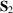,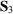and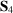(solid rectangles with arrows of spontaneous polarization) formed at a phase transition from a parent phase with symmetry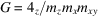to a ferroic phase with symmetry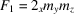(p. 452) | html | pdf |
• Fig. 3.4.2.3. Oriented symmetry operations of the cubic groupand of its subgroups  (p. 460) | html | pdf |
• Fig. 3.4.2.4. Oriented symmetry operations of the hexagonal groupand of its hexagonal and trigonal subgroups  (p. 461) | html | pdf |
• Fig. 3.4.2.5. Four basic single-domain states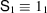,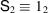,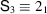,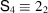of the ferroic phase of a calomel (Hg 2 Cl 2 ) crystal  (p. 469) | html | pdf |
• Fig. 3.4.3.1. Transposable domain pairs  (p. 472) | html | pdf |
• Fig. 3.4.3.2. Non-transposable domain pairs  (p. 472) | html | pdf |
• Fig. 3.4.3.3. Domain structure in lead germanate observed using a polarized-light microscope  (p. 480) | html | pdf |
• Fig. 3.4.3.4. Temperature dependence of lattice parameters in leucite  (p. 481) | html | pdf |
• Fig. 3.4.3.5. Formation of a ferroelastic domain twin  (p. 482) | html | pdf |
• Fig. 3.4.3.6. High-resolution electron microscopy image of a ferroelastic twin in the orthorhombic phase of WO 3   (p. 483) | html | pdf |
• Fig. 3.4.3.7. Ferroelastic twins in a very thin YBa 2 Cu 3 O 7−δ crystal observed in a polarized-light microscope  (p. 484) | html | pdf |
• Fig. 3.4.3.8. Exploded view of four ferroelastic twins with disoriented ferroelastic domain states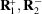and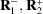formed from a single-domain pair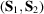(p. 485) | html | pdf |
• Fig. 3.4.3.9. Splitting of diffraction spots from the four domain twins in Fig. 3.4.3.8  (p. 485) | html | pdf |
• Fig. 3.4.3.10. Domain pairs in calomel  (p. 491) | html | pdf |
• Fig. 3.4.4.1. Symbols of a simple twin  (p. 491) | html | pdf |
• Fig. 3.4.4.2. A simple twin under the action of four types of operation that do not change the orientation of the wall plane p   (p. 493) | html | pdf |
• Fig. 3.4.4.3. Engineered periodic non-ferroelastic ferroelectric stripe domain structure within a lithium tantalate crystal with symmetry descent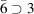(p. 496) | html | pdf |
• Fig. 3.4.4.4. Microscopic structure of two domain states and two parallel mutually reversed domain walls in thephase of quartz  (p. 497) | html | pdf |
• Fig. 3.4.4.5. Transmission electron microscopy (TEM) image of the incommensurate triangular (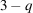modulated) phase of quartz  (p. 497) | html | pdf |
• Fig. 3.4.4.6. Profile of the one-component order parameter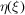in a symmetric wall (S)  (p. 499) | html | pdf |
• Fig. 3.4.4.7. Profiles of the one-component order parameterin a symmetric wall (solid curve) and in the reversed wall (dotted curve)  (p. 500) | html | pdf |
• Fig. 3.4.4.8. Profiles of the one-component order parameterin an asymmetric wall (solid curve) and in the reversed asymmetric wall (dotted curve)  (p. 500) | html | pdf |
• Fig. 3.4.4.9. Profiles of the one-component order parameterin an asymmetric wall (solid curve) and in the reversed asymmetric wall (dotted curve)  (p. 500) | html | pdf |
• Fig. 3.4.4.10. A profile of the second order parameter component in a degenerate domain wall  (p. 501) | html | pdf |
• Fig. 3.4.4.11. Microscopic structure of a ferroelastic domain wall in calomel  (p. 502) | html | pdf |
• Tables
• Table 3.4.2.1. Left and double cosets, principal and secondary domain states and their tensor parameters for the phase transition withand(p. 454) | html | pdf |
• Table 3.4.2.2. Crystal systems, holohedries, crystal families and number of spontaneous strain components  (p. 456) | html | pdf |
• Table 3.4.2.3. Aizu's classification of ferroic phases  (p. 457) | html | pdf |
• Table 3.4.2.4. Morphic properties, tensor parameters, order parameters, stabilizers and domain states  (p. 459) | html | pdf |
• Table 3.4.2.5. Symbols of symmetry operations of the point group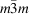(p. 460) | html | pdf |
• Table 3.4.2.6. Symbols of symmetry operations of the point group(p. 461) | html | pdf |
• Table 3.4.2.7. Group–subgroup symmetry descents(pp. 463-467) | html | pdf |
• Table 3.4.3.1. Classification of domain pairs, ferroic phases and of switching (state shifts)  (p. 475) | html | pdf |
• Table 3.4.3.2. Four types of domain pairs  (p. 476) | html | pdf |
• Table 3.4.3.3. Decomposition of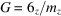into left cosets of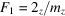(p. 476) | html | pdf |
• Table 3.4.3.4. Non-ferroelastic domain pairs, domain twin laws and distinction of non-ferroelastic domains  (pp. 478-479) | html | pdf |
• Table 3.4.3.5. Property tensors and switching fields  (p. 479) | html | pdf |
• Table 3.4.3.6. Ferroelastic domain pairs and twins with compatible walls  (pp. 486-489) | html | pdf |
• Table 3.4.3.7. Ferroelastic domain pairs with no compatible domain walls  (p. 490) | html | pdf |
• Table 3.4.4.1. Crystallographic layer groups with continuous translations  (p. 492) | html | pdf |
• Table 3.4.4.2. Action of four types of operations g on a twin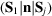(p. 494) | html | pdf |
• Table 3.4.4.3. Classification of domain walls and simple twins  (p. 495) | html | pdf |
• Table 3.4.4.4. Symmetries of non-ferroelastic domain twins and walls  (p. 496) | html | pdf |
• Table 3.4.4.5. Symmetry properties of domain walls in quartz  (p. 497) | html | pdf |
• Table 3.4.4.6. Symmetry properties of ferroelastic domain twins and compatible domain walls  (p. 498) | html | pdf |
• Table 3.4.4.7. Sectional layer groups and twin (wall) symmetries of the twin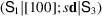in a calomel crystal  (p. 502) | html | pdf |